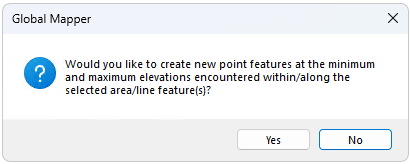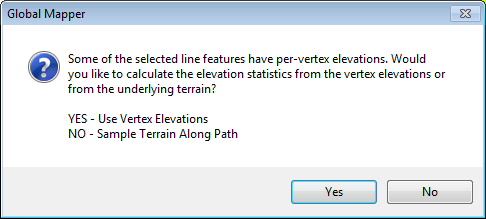# Calculate Elevation / Slope Statistics for Selected Features

This tool will calculate a number of elevation and slope statistics for selected vector

If gridded elevation data (i.e. DEMs) is loaded and at least one feature is selected with the Digitizer tool, the Calculate Elevation/Slope Stats for Selected Feature(s)...option is available in the Digitizer menu or context menu under Analysis/ Measurement or Attribute/ Style Functions.

The Calculate Elevation/Slope Stats for Selected Feature(s)... will also provide an option to create point features at the minimum and maximum elevations of each selected feature.Selecting this option will examine the loaded terrain within the selected area feature(s) and, if terrain is found within a selected area, add MIN_ELEV, MAX_ELEV, AVG_ELEV, STD_DEV_ELEV, MAX_SLOPE, AVG_SLOPE, and STD_DEV_SLOPE attributes to the area containing the minimum, maximum, average, and standard deviation of elevation values encountered within the area feature as well as the maximum, average, and standard deviation of the slope values (in degrees) found within the area.

## Area Statistics

The calculated statistics for area features are as follows:

## Line Statistics

For selected line features, the maximum and average slope (in degrees), the total elevation gain and loss along the path, and the maximum slope both up and down the line (assuming travel from the start of the line to the end) for the line will be computed and added as MAX_SLOPE, MAX_UP_SLOPE, MAX_DOWN_SLOPE, AVG_SLOPE, ELEV_GAIN, and ELEV_LOSS attributes. For selected point features, the ELEVATION, SLOPE, and SLOPE_DIR attributes will be calculated and added for each point that is on a loaded terrain surface.

The line feature statistics may either be sampled along the line based on the underlying terrain, or using only per vertex elevation values. If the line feature is 3D and has per-vertex elevations, an additional dialog will specify whether to sample along the line, or use the per vertex elevations for the calculated elevation statistics.The calculated statistics for line features are as follows:

## Point Statistics

The calculated statistics for point features include: